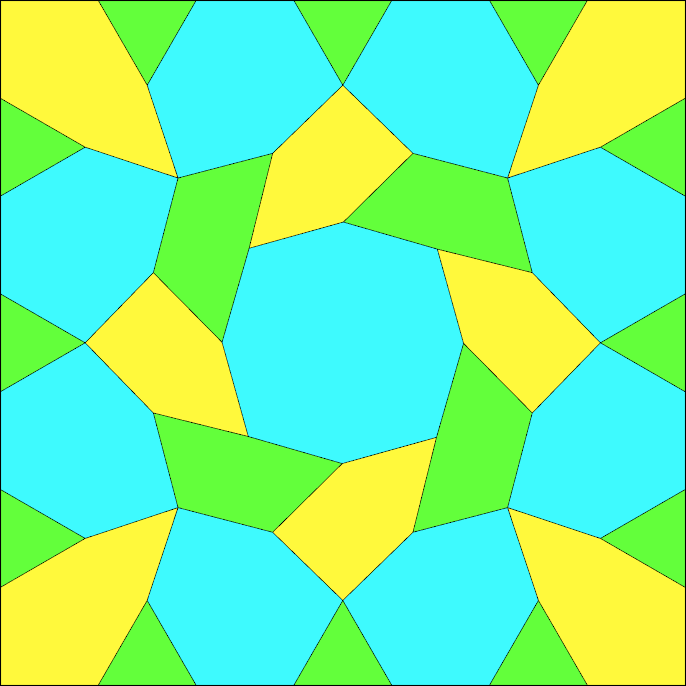I am a CNRS researcher working at Laboratoire Paul Painlevé at the University of Lille.

My research interests include modular forms, algebraic number theory, convex and discrete geometry, combinatorics, as well as approximation theory and Fourier analysis.

## Publications

1. (with J. P. G. Ramos) Sharp Gaussian decay for the one-dimensional harmonic oscillator
Preprint, 2023. arXiv:2305.18546

2. (with K. J. Böröczky and J. P. G. Ramos) A quantitative stability result for the sphere packing problem in dimensions 8 and 24
Preprint, 2023. arXiv:2303.07908

3. (with D. Berghaus and H. Monien) A database of modular forms on noncongruence subgroups
Preprint, 2023. arXiv:2301.02135

4. (with D. Berghaus, R.S. Jones, and H. Monien) Computation of Laplacian eigenvalues of two-dimensional shapes with dihedral symmetry
Preprint, 2022. arXiv:2210.13229

5. (with S. Charlton, H. Gangl, and D. Rudenko) On the Goncharov Depth Conjecture and polylogarithms of depth two
Preprint, 2022. arXiv:2210.11938

6. (with K. Klinger-Logan and S.D. Miller) The D6R4 interaction as a Poincaré series, and a related shifted convolution sum
Preprint, 2022. arXiv:2210.00047

7. (with D. Berghaus and H. Monien) On the computation of modular forms on noncongruence subgroups
Preprint, 2022. arXiv:2207.13365

8. (with A. Arman, A. Bondarenko, and A. Prymak) Upper bounds on chromatic number of 𝔼n in low dimensions
Preprint, 2021. arXiv:2112.13438

9. (with D. Berghaus, B. Georgiev, and H. Monien) On Dirichlet eigenvalues of regular polygons
Preprint, 2021. arXiv:2103.01057

10. (with S. Charlton and H. Gangl) Explicit formulas for Grassmannian polylogarithms
Preprint, 2019. arXiv:1909.13869

11. (with S. Charlton and H. Gangl) Functional equations of polygonal type for multiple polylogarithms in weights 5, 6 and 7
Pure and Applied Mathematics Quarterly 19(1), pp. 85–93 (2023), doi:10.4310/PAMQ.2023.v19.n1.a5
arXiv:2012.09840

12. (with D. Zagier) Arithmetic properties of the Herglotz function
Journal für die reine und angewandte Mathematik, 2023, https://doi.org/10.1515/crelle-2023-0009
arXiv:2012.15805

13. (with H. Cohn, A. Kumar, S.D. Miller, and M. Viazovska) Universal optimality of the E8 and Leech lattices and interpolation formulas
Annals of Mathematics 196(3), pp. 983–1082 (2022)
arXiv:1902.05438

14. (with A. Bondarenko and K. Seip) Fourier interpolation with zeros of zeta and L-functions
Constructive Approximation, Published: 10 Nov. 2022, https://doi.org/10.1007/s00365-022-09599-w
arXiv:2005.02996

15. (with M. Stoller) Fourier non-uniqueness sets from totally real number fields
Comment. Math. Helv. 97 no. 3, pp. 513–553 (2022)
arXiv:2108.11828

16. (with D. Grigoriev) On a tropical version of the Jacobian conjecture
J. Symb. Comput. 109, pp. 399–403 (2022)
arXiv:1902.07733

17. (with A. Bondarenko and A. Prymak) Spherical coverings and X-raying convex bodies of constant width
Canad. Math. Bull. 65(4), pp. 860–866 (2022), doi:10.4153/S0008439521001016
arXiv:2011.06398

18. (with F. Villegas) Independence polynomials and hypergeometric series
Bull. Lond. Math. Soc. 53 (2), pp. 1834–1848 (2021)
arXiv:1908.11231

19. (with S. Charlton and H. Gangl) On functional equations for Nielsen polylogarithms
Comm. N. Th. Phys. 15 (2), pp. 363–454 (2021)
arXiv:1908.04770

20. (with A. Bakan, H. Hedenmalm, A. Montes-Rodriguez, and M. Viazovska) Fourier uniqueness in even dimensions
PNAS 118(15), e2023227118 (2021)
arXiv:2007.03981

21. Unit distance graphs and algebraic integers
Discrete Comput. Geom. 66, pp. 269–272 (2021)
arXiv:1807.03726

22. (with M. Viazovska) Fourier interpolation on the real line
Publ. Math. Inst. Hautes Études Sci. 129, pp. 51–81 (2019)
arXiv:1701.00265

23. (with F. Villegas) Goursat rigid local systems of rank four
Res. Math. Sci. 5:38, in the collection: Modular Forms are Everywhere: Celebration of Don Zagier's 65th Birthday (2018)
arXiv:1803.08379

24. (with A. Bondarenko, A. Mellit, A. Prymak, and M. Viazovska) There is no strongly regular graph with parameters (460,153,32,60)
In: Dick J., Kuo F., Woźniakowski H. (eds) Contemporary Computational Mathematics - A Celebration of the 80th Birthday of Ian Sloan. Springer, Cham (2018)
arXiv:1509.06286

25. (with H. Cohn, A. Kumar, S.D. Miller, and M. Viazovska) The sphere packing problem in dimension 24
Annals of Mathematics 185(3), pp. 1017–1033 (2017)
arXiv:1603.06518

26. (with A. Bondarenko and A. Prymak) Non-existence of (76, 30, 8, 14) strongly regular graph and some structural tools
Linear Algebra Appl. 527, pp. 53–72 (2017)
arXiv:1410.6748

27. Higher cross-ratios and geometric functional equations for polylogarithms
PhD Thesis, Bonn University, 2016. Link

28. (with A.V. Bondarenko and M.S. Viazovska) Well-separated spherical designs
Constructive Approximation, Vol. 41(1), pp. 93–112 (2015)
arXiv:1303.5991

29. Local Maxima of the Potential Energy on Spheres
Ukr. Math. Journal, Vol. 65(10), pp. 1585–1587 (2014)
arXiv:1103.2552

30. (with A.V. Bondarenko and M.S. Viazovska) Optimal asymptotic bounds for spherical designs
Annals of Mathematics 178(2), pp. 443–452 (2013)
arXiv:1009.4407

31. (with I.V. Livinskii) Representations of algebras defined by a multiplicative relation, corresponding to the Dynkin diagrams D4, E6, E7, E8
Ukr. Math. Journal, Vol. 64(12), pp. 1865–1892 (2013)

32. (with A.V. Bondarenko) On a family of strongly regular graphs with λ = 1
Journal of Combinatorial Theory, Series B 103, pp. 521–531 (2013)
arXiv:1201.0383

33. (with A.V. Bondarenko and A. Prymak) On concentrators and related approximation constants
J. Math. Anal. Appl., Vol. 402(1) pp. 234–241 (2013)
arXiv:1404.2161

34. Approximation by Maps with Nonnegative Jacobian
Mat. Zametki 93:2, pp. 263–275 (2013)
arXiv:1010.5448

35. (with D. Leviatan and I.A. Shevchuk) Positive results and counterexamples in comonotone approximation
Constructive Approximation, Vol. 36(2), pp. 243–266 (2012)

36. (with I. Feschenko, L. Radzivilovsky, and M. Tantsiura) Dissecting a brick into bars
Geometriae Dedicata, Vol. 145(1), pp. 159–168 (2010)
arXiv:0809.1883